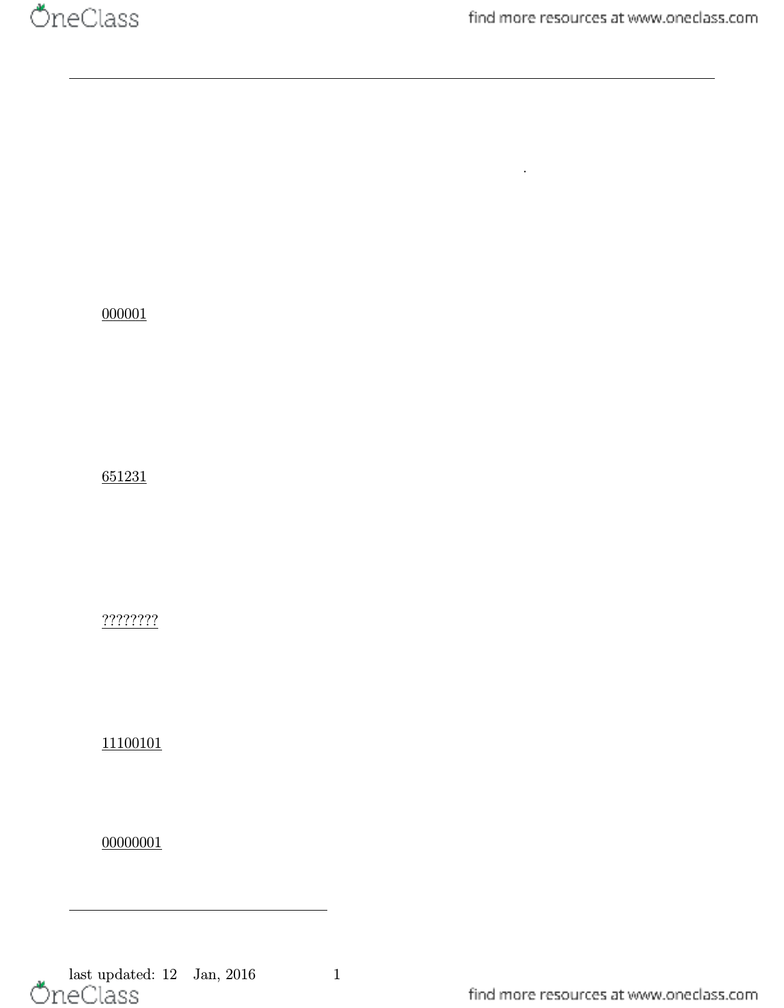Class Notes (1,100,000)
CA (620,000)
McGill (30,000)
COMP (700)
Lecture 1

# COMP 273 Lecture Notes - Lecture 1: Floating Point, Signed Number Representations, Odometer

Department
Computer Science (Sci)
Course Code
COMP 273
Professor
Piotr Przytycki
Lecture
1

Page:
of 7COMP 273 1 - twos complement, ﬂoating point, hexadecimal Jan. 11, 2012
“Twos complement” representation of integers
To motivate our representation of negative numbers, let me take you back to your childhood again.
Remember driving in your parent’s car and looking at the odometer.1Remember the excitement
you felt when the odometer turned from 009999 to 010000. Even more exciting was to see the
odometer go from 999999 to 000000, if you were so lucky. This odometer model turns out to be the
key to how computers represent negative numbers.
If we are working with six digit decimal numbers only, then we might say that 999999 behaves
the same as -1. The reason is that if we add 1 to 999999 then we get 000000 which is zero.
999999
+ 000001
000000
Notice that we are ignoring a seventh digit in the sum (a carry over). We do so because we are
restricting ourselves to six digits.
Take another six digit number 328769. Let’s look for the number that, when added to 328769,
gives us 000000. By inspection, we can determine this number to be 671231.
328769
+ 651231
000000
Thus, on a six digit odometer, 651231 behaves the same as -328769.
Let’s apply the same idea to binary representations of integers. Consider eight bit numbers.
Let’s look at 26ten = 00011010two. How do we represent 26ten ?
00011010
+ ????????
00000000
To ﬁnd 26, we need to ﬁnd the number that, when added to 00011010 gives us 00000000. We use
the following trick. If we “invert” each of the bits of 26 and add it to 26, we get
00011010 26
+ 11100101 inverted bits
11111111
This is not quite right, but its close. We just need to add 1 more. (Remember the odometer).
11111111
+ 00000001
00000000
Note we have thrown away the ninth bit because we are restricting ourself to eight bits only. Thus,
1Ok, ok, odometers were used back in the 20th century, before you were born..
last updated: 12th Jan, 2016 1
COMP 273 1 - twos complement, ﬂoating point, hexadecimal Jan. 11, 2012
00011010 26
11100101 inverted bits
+ 00000001 +1
00000000
Alternatively, we add 1 to the inverted bit representation and this must give us 26.
11100101 inverted bits
+ 00000001 +1
11100110
00011010
11100110 This is 26 10.
00000000
Thus, to represent the negative of a binary number, we invert each of the bits and then add 1. This
is called the twos complement representation.
Special cases
One special case is to check is that the twos complement of 00000000 is indeed 00000000.
00000000
11111111 invert bits
11111111
And adding 1 gets us back to zero. This makes sense, since -0 = 0.
Another special case is the decimal number 128, and again we assume a 8 bit representation. If
you write 128 in 8-bit binary, you get 10000000. Note that taking the twos complement gives you
back the same number 10000000.
10000000
+ 01111111 invert bits
11111111
And adding 1 gets us back to zero. So, what is -128 ?
01111111 the inverted bits
+ 00000001 adding 1
10000000
Note that 128 has the same representation as -128. Of course, we can’t have both: we have to
decide on one. Either 10000000 represents 128 or it represents -128. How does that work?
last updated: 12th Jan, 2016 2
COMP 273 1 - twos complement, ﬂoating point, hexadecimal Jan. 11, 2012
Unsigned vs. signed numbers
If treat all the 8 bit numbers as positive, but we ignore the carry of the leftmost bit in our sum
(the most signiﬁcant bit, or MSB), then adding 1 to the binary number 11111111 (which is 255 in
decimal) takes us back to 0. See the ﬁgure below on the left. This representation is called unsigned.
Unsigned numbers are interpreted as positive.
To allow for negative numbers, we use the twos complement representation. Then we have the
situation of the circle on the right. This is called the signed number representation. Note that the
MSB indicates the sign of the number. If the MSB is 0, then the number is non-negative. If the
MSB is 1, then the number is negative.
00000000
00000001
00000010
11111111
11111110
00000011
10000010
10000001
10000000
01111111
01111110
0
1
2
255
254
3
128
127
126
129
130
0
1
2
-1
-2
3
-128
127
126
- 127
- 126
unsigned signed
Unsigned and signed n-bit numbers
The set of unsigned n-bit numbers is represented on a circle with 2nsteps. The numbers are
{0,1,2,..., 2n1}. It is common to use n= 16,32,64 or 128, though any value of nis
possible. The signed nbit numbers are represented on a circle with 2nsteps, and these numbers are
{− 2n1, . . . , 0,1,2,..., 2n11}. Signed nbit numbers are represented using twos complement.
For example, if n=8, then the signed numbers are {−128,127,..., 0,1,2,..., 127}as we saw
earlier. Consider the following table for the 8 bit numbers.
binary signed unsigned
00000000 0 0
00000001 1 1
: : :
01111111 127 127
10000000 -128 128
10000001 -127 129
: : :
11111111 -1 255
last updated: 12th Jan, 2016 3